# Diffraction

## Introduction

When waves pass through a narrow gap, they spread out. This spreading out is called diffraction. Diffraction is defined as the spreading of a wave into regions where it would not be seen if it moved only in straight lines after passing through a narrow slit or past an edge. Diffraction of a light wave is illustrated in Figure 1.Figure 1: Diffraction

Diffraction may also occur at an edge. When light travels through an aperture or passes the edge of an obstacle, it deviates from the straight-line direction and appears to spread out. Water also exhibits diffraction. The fact that light exhibits diffraction is a proof that light has wave properties. In Figure 2, diffraction is illustrated with two different sizes of aperture. It can be noted that the extent of the diffraction depends on the width of the gap compared with the wavelength. The greatest effect occurs when the wavelength is about the same size as the aperture.Figure 2: Diffraction at Different Size of Aperture

A. Huygens’ Explanation of Diffraction

When a single drop of water falls into a ripple tank, a circular wavefront will spread outwards from the disturbance. This behaviour is explained by Huygens using the wave theory of light. He suggested that, at any instant, all points on a wavefront could be regarded as a secondary disturbance, giving rise to their own outward-spreading circular wavelets. The tangent curve of the wavefronts produced by the secondary sources gives the new position of the original wavefront. The construction of a circular wavefront is shown in Figure 3.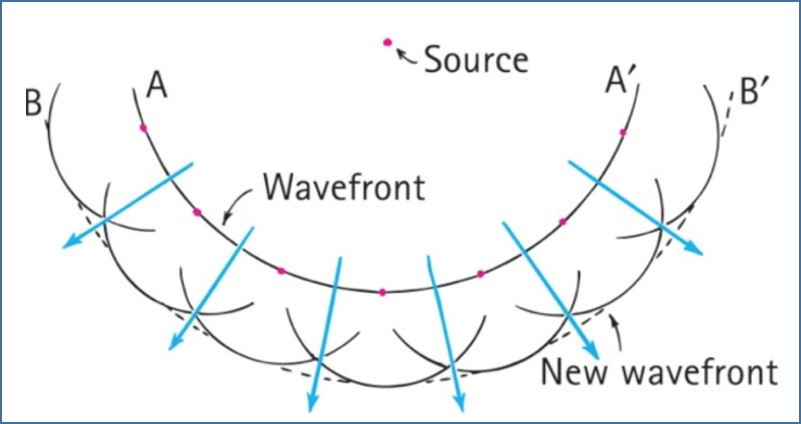Figure 3: Construction of a Circular Wavefront

In the case of a plane wavefront, if it is restricted by passing through an aperture, some of the wavelets forming the wavefront are removed, and hence the edges of the wavefront are curved. In this case, the size of the aperture is larger than the wavelength of the wavefront, the effect of diffraction is relatively small and the wavefront is curved only at the edges. But, if the size of the aperture is approximately equal to the wavelength, the wavefront becomes circular, with the centre on the slit. It can be noted that the wavelength of the wavefront remains the same during diffraction.

B. Diffraction at Single Slit

Figure 4 shows a plane wavefront diffracted at a single slit of width a. Each point on the wavefront passing through the slit can be considered to be a source of secondary wavelets. A is a source at the top edge of the slit and another one is B, at the centre of the slit. B is at a distance a/2 along the wavefront from A. The two sources produce wavelets that spread out and the overlapping points create an interference pattern. Constructive interference creates a bright fringe in the centre of the pattern. The condition for constructive interference is that the path difference should be a whole number of the wavelengths. To either side of the central fringe, there are directions where the path difference between the waves from A and B are an odd number of half-wavelengths. This is called destructive interference and results in dark fringes.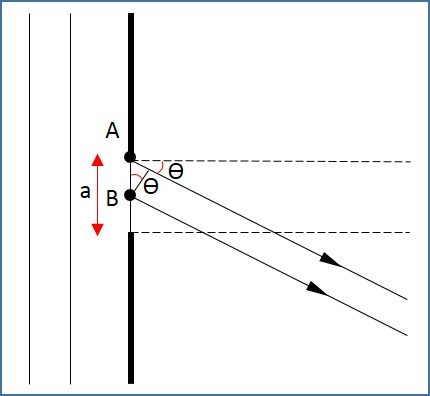Figure 4: Wavefront Diffracted at a Single Slit

The above explanation can be extended to the whole of the slit. Every wavelet spreading from the top half of the slit can be paired with the one coming from a point a/2 below, in the lower half of the slit. Intensity graphs of the diffraction pattern as a function of the angle θ, at which the light is viewed, is shown in Figure 5 below. It can be noted that the intensity is concentrated in the central region. A pattern of dark and bright fringes is also formed along the central region.Figure 5: Diffraction Pattern

For destructive interference,This is the condition for the first dark fringe at angle θ. This can be generalised as,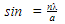Where n is a whole number and is the order of the dark fringe counting outwards from the centre.

For light waves, the width of the aperture is very small and hence the diffraction angle, θ is also very small. When θ is very small (less than 5°),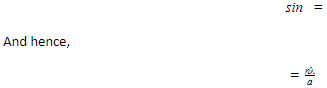This approximation does not apply for sound or water waves, where the diffraction angles are larger and the wavelength may be closer in magnitude to the width of the slit.

C. Diffraction grating

A diffraction grating is a plate on which there is a very large number of parallel, identical, very closely spaced slits. If light is incident on this plate, a pattern of narrow bright fringes is produced, as shown in Figure 6.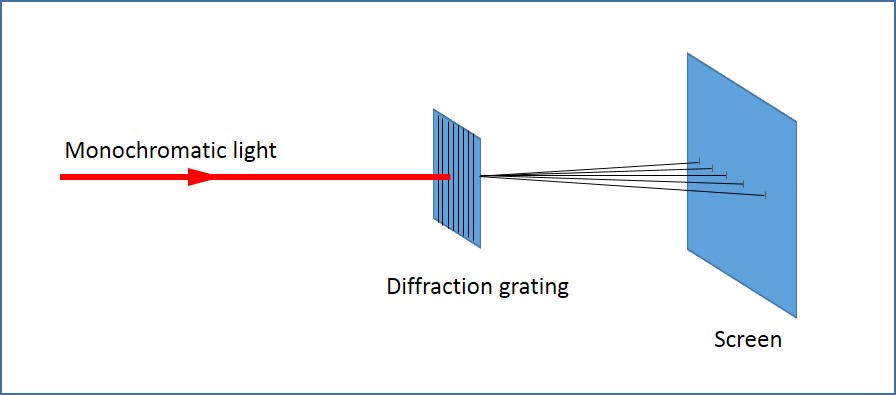Figure 6: Diffraction Grating

Figure 6 shows a parallel beam of monochromatic light incident normally on a diffraction grating, in which the spacing between adjacent slits is d. Let us consider the first rays a and b, incident on adjacent slits as shown in Figure 7.Figure 7: Diffraction Grating

path difference=dsin

Where, θ is the angle at which they emerge.

For constructive interference, the path difference should be an integral number of wavelength,Where λ is the wavelength of the monochromatic light used and n is the integer.

When n=0, sin =0 and hence θ=0, and this is called the zero-order maximum.

When n=1, its called the first-order diffraction maximum, and so on.

D. Summary

• When waves pass through a narrow gap, they spread out. This spreading out is called diffraction.
• Huygens’ explanation of diffraction: At any instant, all points on a wavefront could be regarded as secondary disturbance, giving rise to their own outward-spreading circular wavelets. The tangent curve of the wavefronts produced by the secondary sources gives the new position of the original wavefront.
• In diffraction at a single slit, two sources produce wavelets that spread out and the overlapping points create an interference pattern.
• A diffraction grating is a plate on which there is a very large number of parallel, identical, very closely spaced slits.
• For constructive interference, the path difference should be an integral number of wavelength, d sinӨ=nλ, where n is the order of diffraction.